9.4 C
New York
Saturday, December 2, 2023
HomeChrome OSHow To Calculate Percentage in Google Sheets

# How To Calculate Percentage in Google Sheets

Percent or Percentage, we all are familiar with these words more or less. Actually, there might be no one who is not gotten acquainted with it in their lifetime. However, what is a percentage actually, why is this important, and how can you calculate the percentage in Google Sheets easily? All these questions will be covered in this post.

A percentage provides a clear sense of proportion in the time of expressing quantities. Anyone can make a clear comparison in quantity because of having percentages. Instead of anything in a decimal number, a percent sign can help you to calculate things easily.

However, in our day-to-day life, we use percentages to express many quantities like- bank interest rates, discounts, sales tax, any kind of statistics, and many more. So, in the entire article, I’ll be with you to show how you can calculate percentages in Google Sheets and display them.

## Methods of Calculating Percentage in Google Sheets

Well, before diving into the methods, you need to know one thing. That is, why do you use percentages in Google Sheets?

Honestly speaking, the percentage format is the most effective and popular format in Google Sheets to express values.

However, you can not only just add percentage signs to numbers in Google Sheets but also can convert numbers into percentage values.

### Why Do You Use Percentage in Google Sheets?

Let’s assume you have got a fractional number (0.25) in a cell. Once you convert it into percentage format, it’ll turn into 25%.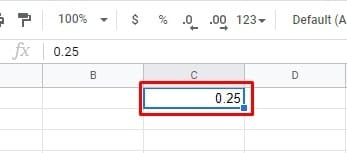Without any doubt, below 25% is more assumable than 0.25. And to do this, you just need to select the cell and click on the % sign from Google Sheets’ top toolbar.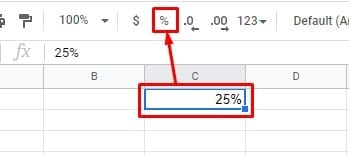However, get back to the question. Why do you need to use percentages? Well, to understand so, let’s take a look at the below images.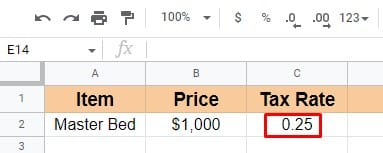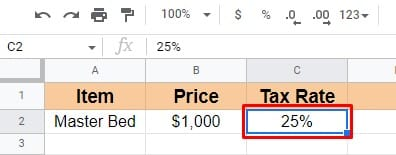As you can see, the same tax rate is being used in both sheets. But, the second one is more understandable than the first one.

It’s happened because of just using the percentage sign in the second one. So, it’s very clear that using percentages is far better than just fractional numbers.

So, I think you would agree with me that the second one is easier to understand than the first example.

Having said that, I’ll be going to discuss three easy methods of how you can calculate percentages in Google Sheets.

However, there might be several situations in which you need to apply percentages. But, I’m going to discuss these below 3 important in particular. Let’s start without any further discussion.

### Method 1: Calculate the Percentage of a Total

In this method, we’ll see how you can calculate the percentage of a total. For example, I’ve prepared sale sheets below based on what each employee has made.

So, I’m going to calculate the total sales made by all the employees together first. To do that, I’ll be using a simple SUM function `=SUM(B2:B9)` to calculate here.

And later on, it will calculate what percentage each employee contributes to the sum of the sales. The formula would be Sales made by each employee/ Total Sales * 100.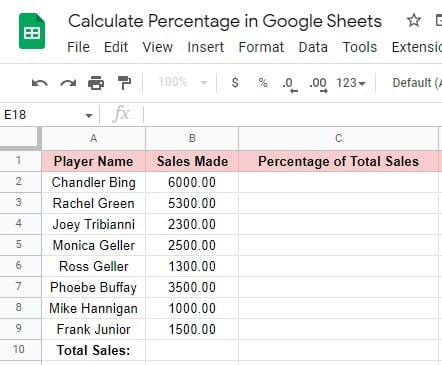#### I. Calculating Total Sales

To calculate the total sales, I clicked on cell B10 and typed the SUM mentioned above function in the cell. Once you’re done typing the formula, hit the Enter button. Thus, you’ll get the total sales.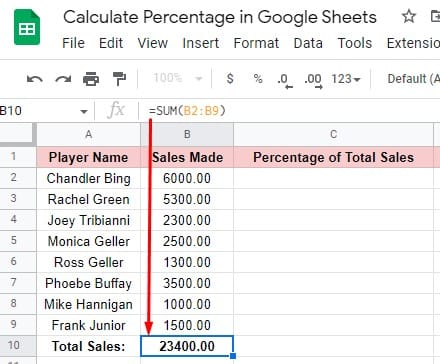#### II. Calculating Sales Percentage Made by Each Employee

Calculating percentages in Google Sheets is super easy. One of them is- calculating the percentage of each individual from a total.

Once you calculate the total sales, you need to follow the below steps to get the percentage of each individual.

Step 1: Select C2 according to my data sheet to get the first row’s percentage result. And type this formula `=B2/\$B\$10` there. Click on Enter key and get the percentage of the first employee has made.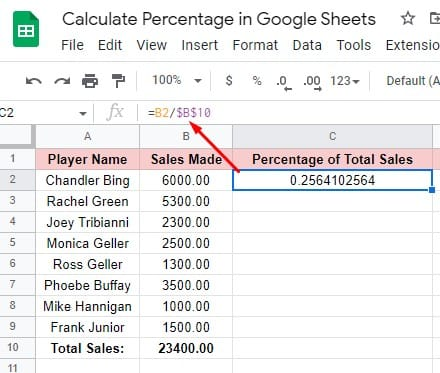Step 2: As you can see above, the percentage result comes with a fractional number. And it’s not very easy to understand.

So to get the number with the % sign, select the cell first and click on the % sign from the top menu bar. As a result, you’ll get a result that is understandable.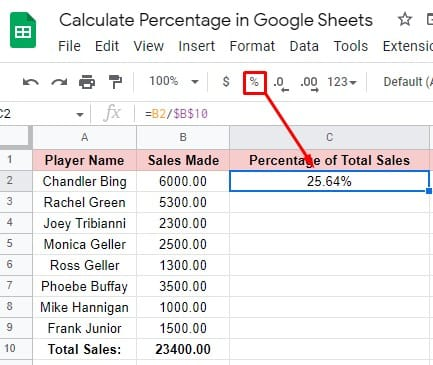Step 3: Now, to get other individual percentages, you don’t need to type the formula into each cell. To get so, select the first cell B2 with the percentage result and drag it from the right corner up to cell B9. And you’ll get all the cell percentage results.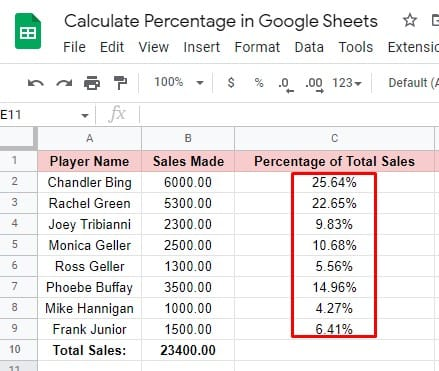Step 4: This is the step to remove the decimal number from the percentage result to make it more concise.

And to do so, select cell C2, and click on the below-marked section twice from the toolbar of your sheets. And you’ll get the actual percentage without any decimal number after it.

However, again drag the cell C2 up to C9 from the below right corner of the cell. You’ll get the percentage format for all the cells eventually.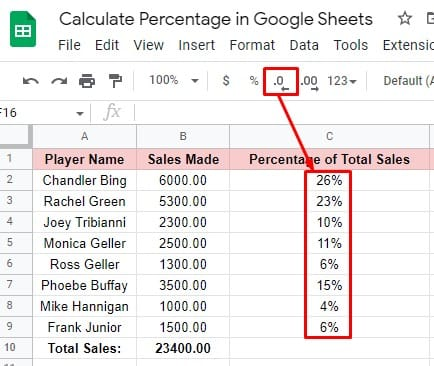This is how you can calculate percentages in Google Sheets from a total.

Apart from this, there might be many situations where you need to have percentages in Google Sheets. Let’s move on to the next methods.

### Method 2: Calculate Percentage in Google Sheets of a Proportion

This is the method of calculating the percentage of a proportion. In simple words, this is the calculation of what portion has been achieved by each individual based on their allocated target.

And, to show you that, I’ve prepared the below datasheet. As you can see, Columns B and C have been classified according to the allotted target of sales and the sales each employee has made during a certain period.

The main goal is to see what percentages they have achieved, which I’m going to show you in Column D. However, the general formula to find this would be: Sales Made / Allotted Target * 100.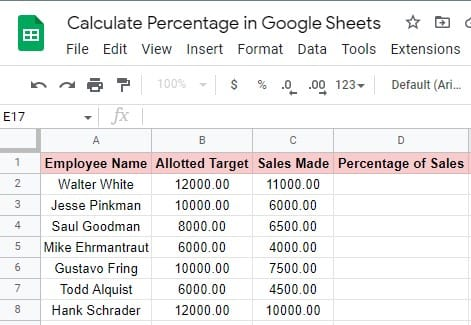#### Calculating Percentages of Each Employee

To apply the above-mentioned general formula in this situation, you need to follow the below steps.

Step 1: As you’ve got the datasheet, now select cell D2 to get percentages of the employee of Row 2. Click on cell D2, and type this formula `=C2/B2` there.

After that, click on Enter key on your keyboard. As a result, you’ll get the percentage as fractional numbers.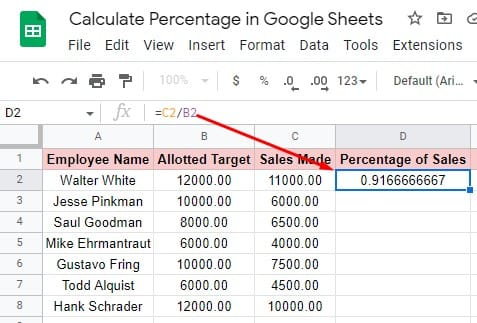Step 2: To convert the fractional numbers into the numbers with the % sign, click on cell D2 and hover over the % icon from the top toolbar. Click on it and get the numbers with the % sign that is easier to understand.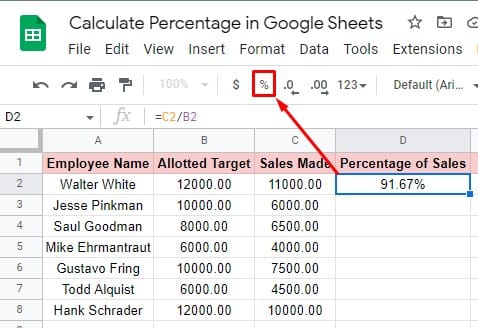Step 3: Drag cell D2 from below the right corner up to cell D8 to get the percentage for the rest of the cells. No need to type the formula into each cell again and again.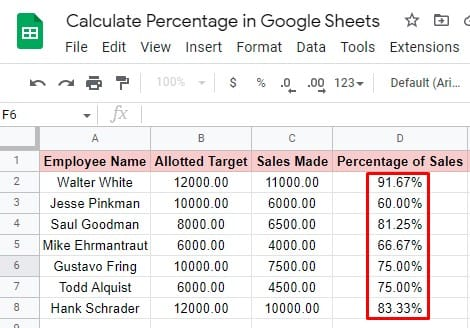Step 4: As you can see in the last image, the percentage still has decimal numbers. However, you can easily get rid of it.

To do so, click on cell D2 or select all the cells (D2:D8), hover over the below-marked section from the toolbar, and click twice on it.

There you are. All the decimal numbers will vanish instantly, and you’ll get the percentage format that you’re looking for. This is how you can calculate percentages in Google Sheets of a proportion.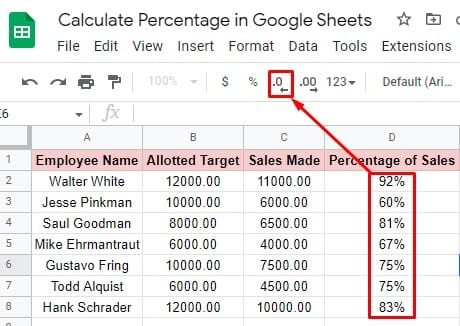### Method 3: Calculate Percentage Change in Google Sheets

In this method, I’ll show you how you can monitor employees’ sales performance. In simple words, how you can calculate percentage changes. This is how you can evaluate employee performance.

In the below datasheet, I put each employee’s two weeks’ sales data in columns B and C. And D to see the evaluation of employees. Simply put, how are they doing with the sales target?

However, to calculate this, the general formula would be (New Value – Old value) / Old value * 100.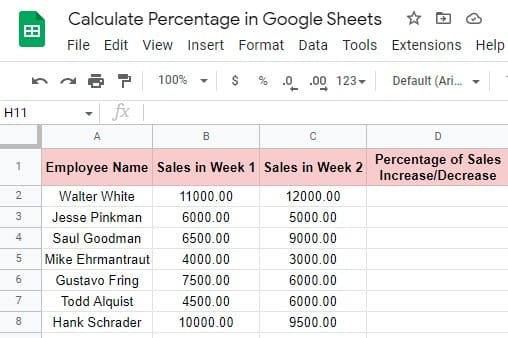And to imply this formula in your datasheets, you need to follow some basic steps to get the result. Here’s the step below.

Step 1: Click on cell D2 to get the row 2 employee result. As follows, type this formula `=(C2-B2)/B2` into the cell. Hit the Enter key and get the percentage result.

However, you can see that the result comes as a fractional number that is hard to understand. So, to get the percentage of the number with the % icon, see the next step.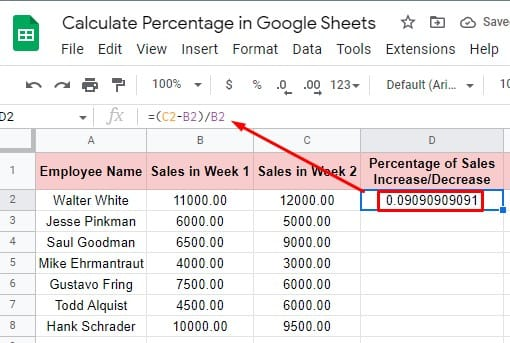Step 2: Clik on cell D2 and head towards the top menu bar. Click on the % icon from there, and get the result you’re seeking for. Now it’s more understandable than before.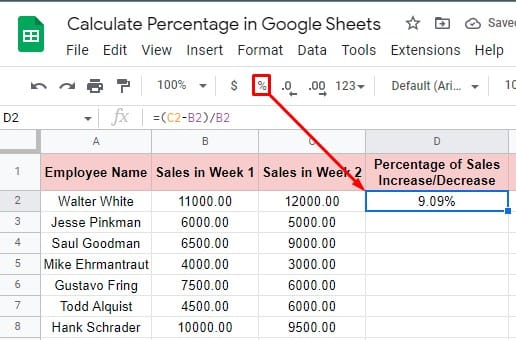Step 3: To get the percentage result of the rest of the employees’ drag cell D2 from below right up to cell D8. And, you’ll have your percentage result.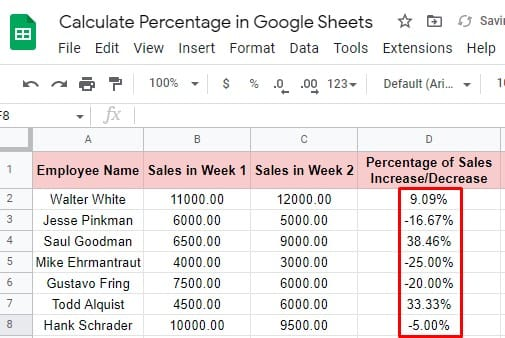Step 4: To remove the decimal numbers from the percentage result, select the cells (D2:D8) and click on the below-marked section from the toolbar.

Here’s the ultimate percentage result from which you can easily evaluate your employees’ sales performance. As you can see, many of them have increased their sales performance; whereas many of them dropped a bit or more than a bit.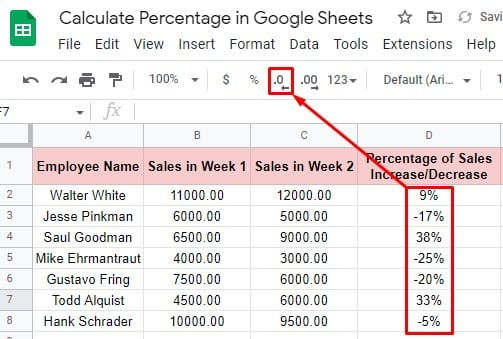## Wrapping Things Up

It’s a wrap. I hope you understood every method mentioned above very clearly. If so, then you can calculate percentages in Google Sheets very easily. Whatever the situation will be, you can calculate the percentage out of that if you went through the methods with concentration.

However, that’s where the post ends. Hoping that you’ll get benefit out of it. So, if you find it helpful and worth sharing, please share so that others can benefit from this. Most importantly, let me know your experience with this in the comment section below.Mehedi Hasan
Mehedi Hasan is a passionate enthusiast for technology. He admires all things tech and loves to help others understand the fundamentals of Linux, servers, networking, and computer security in an understandable way without overwhelming beginners. His articles are carefully crafted with this goal in mind - making complex topics more accessible.